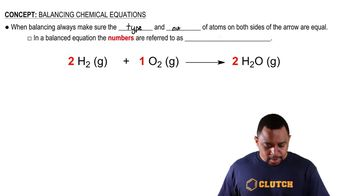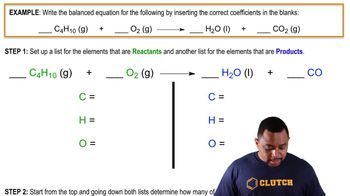Start typing, then use the up and down arrows to select an option from the list.
3:03 minutes
Problem 146
Textbook Question

# When a gaseous compound X containing only C, H, and O is burned in O2, 1 volume of the unknown gas reacts with 3 volumes of O2 to give 2 volumes of CO2 and 3 volumes of gaseous H2O. Assume all volumes are measured at the same temperature and pressure. (a) Calculate a formula for the unknown gas, and write a balanced equation for the combustion reaction.Verified Solution
This video solution was recommended by our tutors as helpful for the problem above.
81views